• c语言实现中缀表达式转后缀表达式并求得计算结果，用顺序栈结构。 当输入者输入错误信息的时候需要报错，并说明错误的种类。
• 初学数据结构，刚接触链表和栈，看到有中缀表达式转后缀的题就试着实现了一下。下面贴上代码。因为使用的是字符型变量，所以只能计算个位数。 /* 堆栈练习——中缀表达式转后缀表达式 */ #include #include #include...
由于是初学时写的代码，所以结构比较混乱
重构后的代码在这里，供参考：http://blog.csdn.net/wait_nothing_alone/article/details/70184819

大一菜鸟，初学编程，这是我的第一篇博客，希望能用博客记录我的成长之路。
初学数据结构，刚接触链表和栈，看到有中缀表达式转后缀的题就试着实现了一下。下面贴上代码。因为使用的是字符型变量，所以只能计算个位数。


/* 堆栈练习——中缀表达式转后缀表达式 */
#include<stdio.h>
#include<stdlib.h>
#include<string.h>
#include<stdbool.h>
#define MAX 25

typedef struct node{
char date;
struct node *next;

typedef struct node2{
float date;
struct node *next;
} Stake;

typedef struct body{
char date[MAX];
int j;
} Body;

void Push(char fuhao, LinkStake *s, Body *p);//压栈
int Judge(bool flag, char c);//判断优先级

{
s=malloc(sizeof(struct node));
s->next = NULL;
return s;
}

{
return(s->next == NULL);
}

void Push(char fuhao, LinkStake *s, Body *p)
{
while(s->next != NULL && Judge(false,fuhao)<Judge(true,s->next->date)){
printf("%c", s->next->date);
p->date[p->j] = s->next->date;
p->j++;
t = s->next;
s->next = t->next;
free(t);
}
if(IsEmpty(s)){
tmp = malloc(sizeof(struct node));
tmp->date = fuhao;
tmp->next = s->next;
s->next = tmp;
}
else if(Judge(false,fuhao)>Judge(true,s->next->date)){
tmp = malloc(sizeof(struct node));
tmp->date = fuhao;
tmp->next = s->next;
s->next = tmp;
}
else if(Judge(false,fuhao) == Judge(true,s->next->date)){
t = s->next;
s->next = t->next;
free(t);
}
}

int Judge(bool flag, char c)
{
if(c == '+' || c == '-')
if(flag) return 3;
else return 2;
else if(c == '*' || c == '/')
if(flag) return 5;
else return 4;
else if(c == '(')
if(flag) return 1;
else return 6;
else if(c == ')')
if(flag) return 6;
else return 1;
else return 0;
}

{
Stake *tmp;
tmp = malloc(sizeof(struct node2));
tmp->date = c;
tmp->next = s->next;
s->next = tmp;
}

{
Stake *t;
float c;
t = s->next;
c = t->date;
s->next = t->next;
free(t);
return c;
}

int main()
{
int i = 0;
Body *p = malloc(sizeof(struct body));
char s[MAX];
p->j = 0;

printf("请输入中缀表达式；\n");
gets(s);
printf("转换为后缀表达式: \n");
while(s[i]){
if(s[i]>='0' && s[i]<='9'){
printf("%c", s[i]);
p->date[p->j] = s[i];
p->j++;
}
i++;
}
p->j++;
free(t);
}
printf("\n");
printf("计算结果为：\n");

Stake *stake;
float sum = 0.0, a, b;
stake = (Stake*)malloc(sizeof(struct node2));
stake->next = NULL;
for(int i = 0; i < p->j; i++){
if(p->date[i]>='0' && p->date[i]<='9')
PushStake((float)(p->date[i]-'0'), stake);
else if(p->date[i]=='+'){
b = PopStake(stake);
a = PopStake(stake);
sum = a+b;
PushStake(sum, stake);
}
else if(p->date[i]=='-'){
b = PopStake(stake);
a = PopStake(stake);
sum = a-b;
PushStake(sum, stake);
}
else if(p->date[i]=='*'){
b = PopStake(stake);
a = PopStake(stake);
sum = a*b;
PushStake(sum, stake);
}
else if(p->date[i]=='/'){
b = PopStake(stake);
a = PopStake(stake);
sum = a/b;
PushStake(sum, stake);
}
}
printf("%.2f", sum);
return 0;
}
展开全文数据结构
• 表达式的值是栈的经典操作，我只简单地实现了正数的四则运算，包括括号运算，也可计算小数，没有检查表达式是否输入正确，以后再改进。实现过程中借鉴了其它博主的一些算法，如有侵权，我会立即删除。 #include &...
何为中缀表达式
所谓中缀表达式，就是平时看到的正常表达式，带有括号。如1+2*（3+4）
计算中缀表达式的值
对我们而言，很容易就计算出中缀表达式的值，对计算机而言，将中缀表达式转化为前缀和后缀表达式更为容易求值。（博主没有转化，直接利用中缀表达式求值）
为了方便，这里，我们不考虑输入的数为负数的情况，没有检查输入的是否是一个正确的中缀表达式。只简单涉及四则运算和括号，输入的数可以是小数。
思路：

使用两个栈，一个存放操作数，另一个存放操作符
将表达式存入两个栈中，边存边计算
从左往右扫描表达式，遇到操作数则直接将其放入操作数栈。
遇到操作符时，如果优先级低于或等于栈顶操作符优先级，则从操作数栈弹出两个元素进行计算，并将计算结果存入操作数栈，继续与栈顶操作符的比较优先级。
如果遇到操作符高于栈顶操作符优先级，则直接入操作符栈 ；遇到左括号，直接入操作符栈，遇到右括号，则直接出栈并计算，直到遇到左括号。
循环步骤3，直到表达式全部被扫描；若扫描完成后，操作符栈不为空，则依次将操作符栈的元素出栈，同时将操作数里面的元素出栈进行计算，同样将计算结果存入操作数栈，直到操作符栈为空，最后在操作数栈的栈顶元素就是表达式的最终计算结果。

实现效果：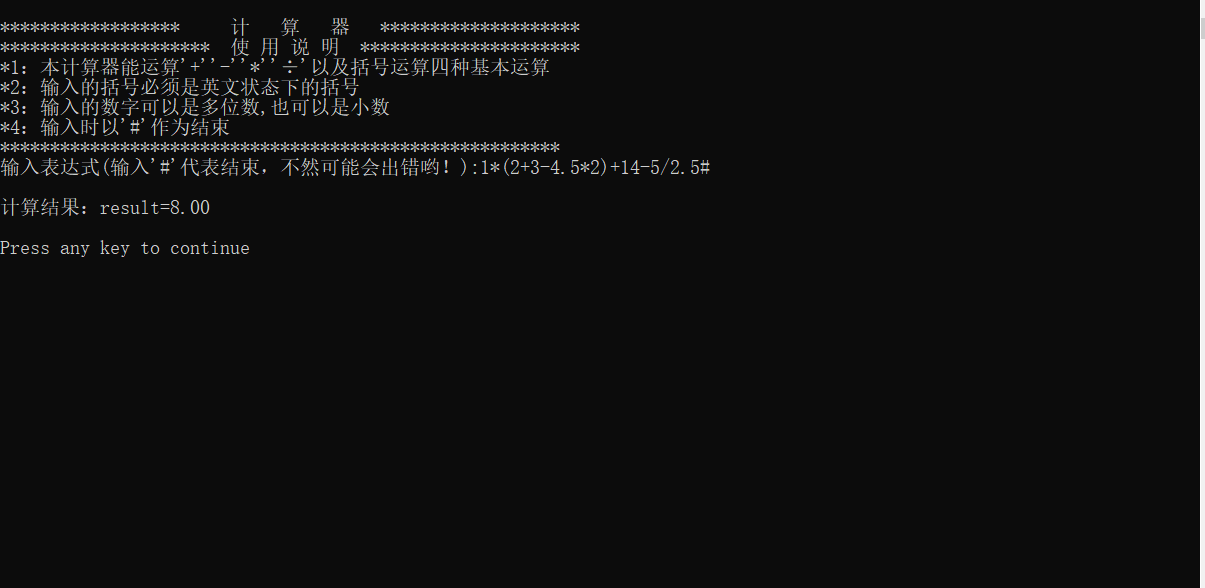代码如下：
#include <stdio.h>
#include <stdlib.h>
typedef struct//操作数栈
{
double *top;
double *base;
int listsize;
}List;
typedef struct//操作符栈
{
char *top;
char *base;
int nodesize;
}Node;
int InitList(List *p)//初始化操作数栈
{
p->base=(double *)malloc(100*sizeof(double));
if(!p->base)
return 0;
p->top=p->base;
p->listsize=100;
return 1;
}
int PushList(List *p,double e)//操作数进栈
{
if(p->top-p->base>=p->listsize)
{
p->base=realloc(p->base,(p->listsize+10)*sizeof(double));
if(!p->base)
return 0;
p->top=p->base+p->listsize;
p->listsize+=10;
}
*(p->top++)=e;
return 1;
}
double PopList(List *p)//操作数出栈
{
double e;
if(p->base==p->top)
return 0;
else
e=*(--p->top);
return e;
}
double getTopList(List *p)//得到操作数栈的栈顶元素
{
double e;
if(p->base==p->top)
return 0;
e=*(p->top-1);
return e;
}
void print(List *p)//打印操作数中的元素
{
double *p1;
if(p->base==p->top)
{
printf("数字空栈！！！！\n");
exit(0);
}
p1=p->top;
while(p1!=p->base)
{
printf("%.2lf\n",*(p1-1));
p1--;
}
}
void printNode(Node *p)//打印操作符中的元素
{
char *p1;
if(p->base==p->top)
{
printf("字符空栈！！！！\n");
exit(0);
}
p1=p->top;
while(p1!=p->base)
{
printf("%c\n\n",*(p1-1));
p1--;
}
}

int InitNode(Node *p)//初始化操作符栈
{
p->base=(char *)malloc(100*sizeof(char));
if(!p->base)
return 0;
p->top=p->base;
p->nodesize=100;
return 1;
}
int PushNode(Node *p,char e)//操作符进栈
{
if(p->top-p->base>=p->nodesize)
{
p->base=realloc(p->base,(p->nodesize+10)*sizeof(char));
if(!p->base)
return 0;
p->top=p->base+p->nodesize;
p->nodesize+=10;
}
*(p->top++)=e;
return 1;
}
char PopNode(Node *p)//操作符出栈
{
char e;
if(p->base==p->top)
return 0;
else
e=*(--p->top);
return e;
}
char getTopNode(Node *p)//得到操作符栈顶元素
{
char e;
if(p->base==p->top)
exit(0);
e=*(p->top-1);
return e;
}
int Length(char *s)//求表达式的长度
{
int i=0,b=0;
while(*(s+i)!='\0')
{
b++;
i++;
}
return b;
}
int empty(Node *p)//判断操作符栈是否为空
{
if(p->base==p->top)
{
return 1;
}
else
return 0;
return 1;
}
int compare(char ch)//判断符号优先级
{
if(ch=='(')return 1;
else if(ch=='#') return 0;
else if(ch=='+'||ch=='-')return 2;
else if(ch=='*'||ch=='/')return 3;
else return 4;
}
{
double c;
switch(op)
{
case '+':c=num2+num1;break;
case '-':c=num2-num1;break;
case '*':c=num2*num1;break;
case '/':c=num2/(num1*1.0);break;
}
return c;
}
int isop(char c)//判断表达式是否符合四则运算
{
if(c=='+'||c=='-'||c=='*'||c=='/'||c=='#')
return 1;
if(c=='('||c==')')
return 2;
/*	else
printf("表达式有误！！！\n");*/
return 0;
}
double calculate()
{
double a,result=0,x,y,b;//a储存进栈的操作数，result为计算结果，x和y是从操作数栈取出的两个栈顶元素
int i=0,j=0,k=0;//i，j为循环变量
char ch,s;//ch是操作符栈顶元素，s为表达式
Node p1;//操作符栈
List p2;//操作数栈
InitList(&p2);
InitNode(&p1);
printf("输入表达式(输入'#'代表结束，不然可能会出错哟！):");
gets(s);
//printf("长度:%d\n",Length(s));
while(s[i]!='\0'&&i<Length(s))
{
k=0;
b=0;
a=0;
j=i;
if(s[j]>='0'&&s[j]<='9')//若是操作数
{

while(s[j]>='0'&&s[j]<='9')       //将操作数（可能有多个连续的数字）转化为double型数据

{
a=(double)((s[j]-'0')+a*10);
j++;
}
if(s[j]=='.')//如果存在小数
{
j++;
if(s[j]>='0'&&s[j]<='9')//若是操作数
{

while(s[j]>='0'&&s[j]<='9')       //将操作数（可能有多个连续的数字）转化为double型数据
{
b=(double)((s[j]-'0')+b*10);
k++;
j++;
}
for(i=0;i<k;i++)
b=b/(10.0);
a=a+b;
}
}

i=--j;
PushList(&p2,a);
}

else if(isop(s[i])==1)//若是+，-，*，/
{
if(empty(&p1)) //操作符栈为空，直接进栈
PushNode(&p1,s[i]);
else//操作符栈不为空
{
while(!empty(&p1))
{
ch=getTopNode(&p1);//取栈顶操作符
//printf("ch=%c\n",ch);
if(compare(ch)>=compare(s[i]))//当前即将进栈的元素大于栈顶元素的优先级，则计算
{
x=PopList(&p2);
y=PopList(&p2);
//	printf("结果:%.2lf\n",result);
PopNode(&p1);
PushList(&p2,result);
}
else break;
}
if(s[i]!='#')
PushNode(&p1,s[i]);
}
//i++;
}
else if(isop(s[i])==2)//若有括号即将进栈
{
if(s[i]=='(')
PushNode(&p1,s[i]);//左括号直接进栈
else//若为右括号
{
while(getTopNode(&p1)!='(')//直到找到左括号，否则计算
{
ch=getTopNode(&p1);
x=PopList(&p2);
y=PopList(&p2);
PopNode(&p1);
PushList(&p2,result);
}
PopNode(&p1);
}
}
i++;
}
while(!empty(&p1))//若操作符栈不为空，则继续计算
{
ch=getTopNode(&p1);
x=PopList(&p2);
y=PopList(&p2);
PopNode(&p1);
PushList(&p2,result);
}
//	return getTopList(&p2);
//	print(&p2);
//	printNode(&p1);
return getTopList(&p2);//返回操作数的栈顶元素（即为最终计算结果）
}

int main()
{
printf("\n******************     计   算   器   ********************\n");
printf("*********************  使 用 说 明  **********************\n");
printf("*1：本计算器能运算'+''-''*''÷'以及括号运算四种基本运算\n");
printf("*2：输入的括号必须是英文状态下的括号\n");
printf("*3：输入的数字可以是多位数,也可以是小数\n");
printf("*4：输入时以'#'作为结束\n");
printf("********************************************************\n");
printf("\n计算结果：result=%.2lf\n\n",calculate());
return 0;
}




展开全文• //用于指向字符串(中缀表达式)的指针 //声明两个栈，一个float型，一个char型 //表达式中操作数存在一个栈内，运算符存入一个栈内 //top1,top2用于指向两个栈的栈顶 float s1[maxSize];int top1=-1; char ...
#include<stdio.h>
#include<stdlib.h>
#include<string.h>
#include<math.h>
#define Min 1e-8
#define maxSize 10
int priority(char p){
if(p=='+'||p=='-'){
return 0;
}else{
return 1;
}
}
//子表达的计算
int calSub(float opand1,char op,float opand2,float &result){
//根据操作符进行计算
if(op == '*') result=opand1*opand2;
if(op == '+') result=opand1+opand2;
if(op == '-') result=opand1-opand2;
if(op == '/'){//若是除则需要判断除数为零的情况
if(fabs(opand2)<Min){// 浮点数没有准确的表达，只能用绝对值来表示差不多的范围
return 0;
}
else{
result =opand1/opand2;
}
}
return 1;
}
int calStackTopTwo(float s1[],int &top1,char s2[],int &top2){
float a=s1[top1--];//弹出栈顶元素后指针下移
float b=s1[top1--];
float result;
char op=s2[top2--];
int flag=calSub(b,op,a,result);//进行子表达式的计算
if(flag==0){
printf("被除数为零");
return 0;
}
s1[++top1]=result;//计算结果存入栈顶
return 1;

}
//计算表达式的函数
float getResult(char exp[]){
int i=0;//用于指向字符串(中缀表达式)的指针
//声明两个栈，一个float型，一个char型
//表达式中操作数存在一个栈内，运算符存入一个栈内
//top1,top2用于指向两个栈的栈顶
float s1[maxSize];int top1=-1;
char s2[maxSize];int top2=-1;

while(exp[i]!='\0'){//字符串结尾以'\0'
if(exp[i]>='0'&&exp[i]<='9'){//判断是否是数字，但此处只能判断0-9之间的一位数字
s1[++top1]=exp[i]-'0';//字符串转换为数字
++i;//下一个字符
}
else if(exp[i]=='(') {//如果是左括号的话直接进栈
s2[++top2]='(';
i++;
}else if(exp[i]=='*'|| //如果是运算符则进行以下判断
exp[i]=='/'||
exp[i]=='+'||
exp[i]=='-'){
//如果栈顶为左括号或者栈空，或者当前符号优先级大于栈顶符号优先级则入栈
if(s2[top2]=='('||top2==-1||priority(exp[i])>priority(s2[top2])){
s2[++top2]=exp[i];
i++;
}else{
int flag=calStackTopTwo(s1,top1,s2,top2);//当前运算符小于栈顶元素优先级则弹出数据栈的两个
if(flag==0){
return 0;
}
}                                  //操作数，进行运算后存入栈顶，完毕后i的值不发生
}                                      //变化
else if(exp[i]==')'){//如果碰到右括号，则弹出栈内直至左括号的所有运算符
while(s2[top2]!='('){//弹出一个运算符都需要进行计算
int flag=calStackTopTwo(s1,top1,s2,top2);
if(flag==0){
return 0;
}

}
--top2;//更新栈顶指针
i++;

}

}
//当表达式进栈完毕后
//只要符号栈内还有元素就继续进行运算
while(top2!=-1){
int flag=calStackTopTwo(s1,top1,s2,top2);
if(flag==0){
return 0;
}
}
return s1[top1];
}
//后缀表达式计算
float calPostFix(char exp[]){
float s1[maxSize];
int top=-1;
int i=0;
while(exp[i]!='\0'){
if(exp[i]>='0'&&exp[i]<='9'){
s1[++top]=exp[i]-'0';
}else{
float a,b,result;
a=s1[top--];
b=s1[top--];//出栈顶的两个元素
int flag=calSub(a,exp[i],b,result);//子表达式计算结果存入result
if(flag==0){
printf("Error");
return 0;
}
s1[++top]=result;//将结果存入栈顶
}
i++;

}
return s1[top];

}
float calPreFix(char exp[],int length){
float s1[maxSize];
int top=-1;
for(int i=length-1;i>=0;--i){
if(exp[i] >= '0' && exp[i]<='9'){
//printf("%c",exp[i]);
s1[++top]=exp[i]-'0';
}else
{
float a,b,result;
a=s1[top--];
b=s1[top--];//出栈顶的两个元素
//	printf("%f %f",s1,s1);
int flag=calSub(a,exp[i],b,result);//子表达式计算结果存入result
if(flag==0){
printf("Error");
return 0;
}
s1[++top]=result;//将结果存入栈顶
}
}
return s1[top];
}
//中缀式转后缀式
//参数top传引用型是为了能够查看栈的最大用量
//参数s2[]数组是存储结果表达式
void infixtoPostFix(char infix[],char s2[],int &top2){
char s1[maxSize];//辅助栈
int i=0,top1=-1;
while(infix[i]!='\0'){

if(infix[i]<='9'&&infix[i]>='0'){
s2[++top2]=infix[i];
i++;
}else if(infix[i]=='('){
s1[++top1]=infix[i];

i++;
}else if(infix[i]=='*'||infix[i]=='+'||infix[i]=='-'||infix[i]=='/'){
if(top1==-1||s1[top1]=='('||priority(infix[i])>priority(s1[top1])){
//中缀转后缀，当前运算符优先级小于等于栈顶运算符优先级则出栈
s1[++top1]=infix[i];
i++;
}else{
s2[++top2]=s1[top1--];
}
}else if(infix[i]==')'){
while(s1[top1]!='('){
s2[++top2]=s1[top1--];

}
--top1;//
i++;
}
}
while(top1!=-1){
s2[++top2]=s1[top1--];

}
}
//中缀式转前缀式
void infixtoPreFix(char infix[],int length,char s3[],int &top2){
char s1[maxSize];//辅助栈
char s2[maxSize];//
int i=length-1,top1=-1;
while(i>=0){
if(infix[i]<='9'&&infix[i]>='0'){
s2[++top2]=infix[i];
i--;
}else if(infix[i]==')'){
s1[++top1]=infix[i];
i--;
}else if(infix[i]=='*'||infix[i]=='+'||infix[i]=='-'||infix[i]=='/'){
//中缀转前缀，当前运算符优先级小于栈顶运算符优先级则出栈
if(top1==-1||s1[top1]==')'||priority(infix[i]) >= priority(s1[top1])){
s1[++top1]=infix[i];
i--;
}else{
s2[++top2]=s1[top1--];
}
}else if(infix[i]=='('){
while(s1[top1]!=')'){
s2[++top2]=s1[top1--];
}
--top1;
i--;
}
}
while(top1!= -1){
s2[++top2]=s1[top1--];
}
int v=0;
for(int i=top2;i>=0;i--){

s3[v++]=s2[i];
}

}
int getlength(char s[]){
int length=0;
for(int i=0;i<maxSize;i++){
if((s[i]<'9'&&s[i]>'0')||s[i]=='+'||s[i]=='*'||
s[i]=='/'||s[i]=='-'||s[i]=='('||s[i]==')'){
length++;
}
}
return length;
}
int main(){
char s[maxSize];
printf("请输入中缀表达式：\n");
scanf("%s",s);//录入字符串
printf("%s=%f\n",s,getResult(s));

char result[maxSize];//存放转换后结果
int top1=-1;
infixtoPostFix(s,result,top1);
printf("中缀转后缀为：%s\n",result);
printf("后缀的结果表达式为：%f\n",calPostFix(result));
int top2=-1;
char result1[maxSize];//存放转换后结果

infixtoPreFix(s,getlength(s),result1,top2);
printf("中缀转前缀为：%s\n",result1);
printf("前缀的结果表达式为：%f\n",calPreFix(result1,getlength(s)));

}

代码运行截图：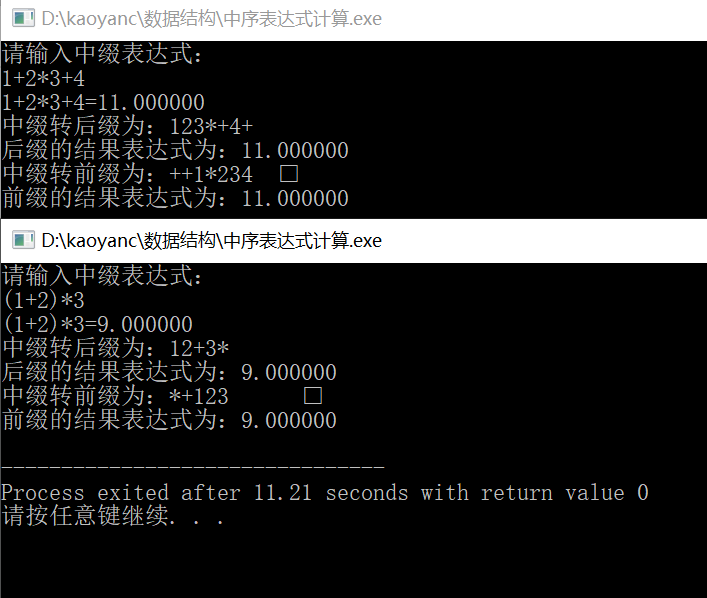其中在转化时需要注意：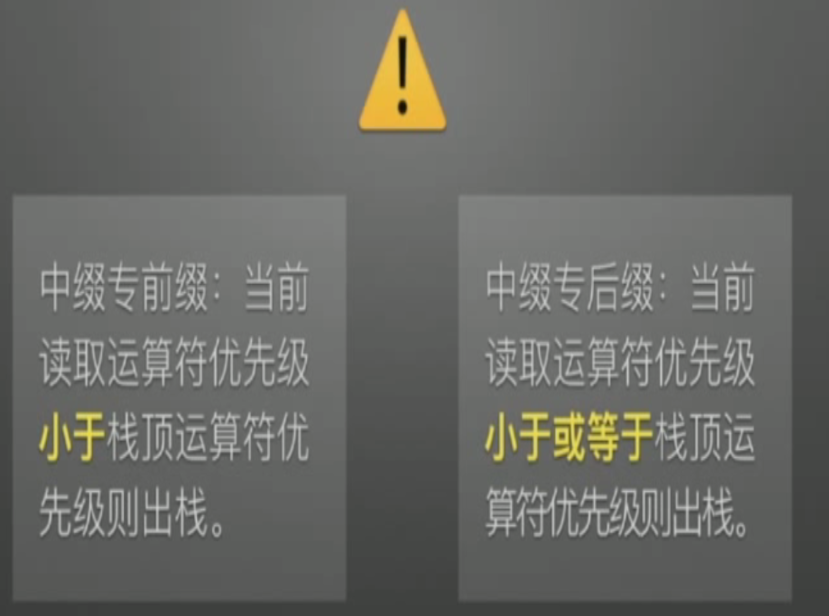展开全文• 接下来就为大家讲解一下这份由iMindMap制作的C语言表达式思维导图。C语言表达式一共有五块内容。一、算术运算符顾名思义，算术运算符就是我们在进行算式计算时使用到的运算符。图片1：算术运算符在C语言中，根据参与...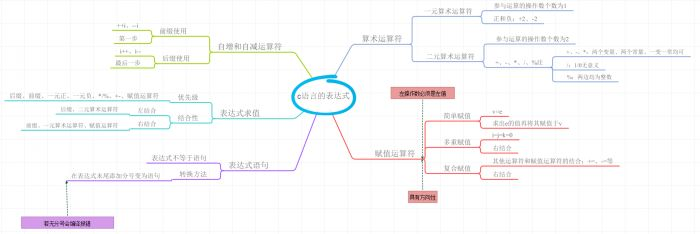思维导图是我们在学习和了解事物，尤其是新知识时的一个好工具。比如最近我在学习的C语言课程，知识点零碎且乱，但使用思维导图就可以简单直白地总结这些零碎的内容。接下来就为大家讲解一下这份由iMindMap制作的C语言表达式思维导图。C语言表达式一共有五块内容。一、算术运算符顾名思义，算术运算符就是我们在进行算式计算时使用到的运算符。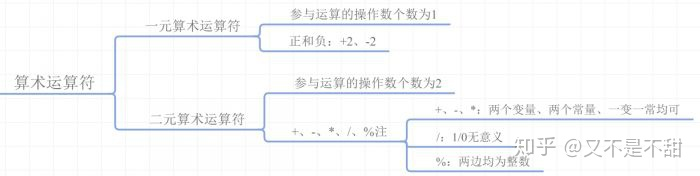图片1：算术运算符在C语言中，根据参与运算的操作数个数分为一元运算符和二元运算符，表示参与运算的有一个或两个操作数。一元算术运算符就是在数值前面带上正号或负号；二元算术运算符就是我们常说的加减乘除以及除数取余。一元运算符不常使用，二元运算符在使用过程中各有各的注意事项，在导图中可以清晰地列出来。二、赋值运算符赋值运算符就是将一个数赋值给另一个数，这里要注意的是这里的表达式具有方向性，即右边赋于左边，并且左操作数必须是左值。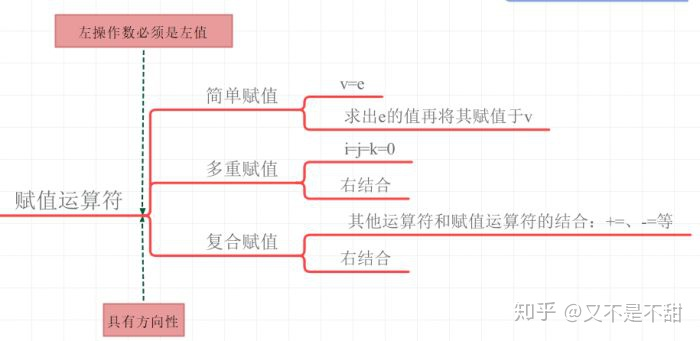图片2：赋值运算符赋值包括简单赋值、多重赋值和复合赋值。在简单赋值v=e这个表达式中，实际包含两个步骤，首先是将e的值计算出来，然后是将该值赋予v。多重赋值属于右结合运算，即从右侧开始逐一向左侧赋值。复合赋值则是将其他类型的运算符，比如算术运算符，与简单赋值运算符结合使用。三、自增和自减运算符自增和自减运算是指在数值本身的基础上加一或减一的运算，每个运算符可以进行一次加一或减一操作。图片3：自增和自减运算符其中包括后缀和前缀两种形式，它们的主要区别在于后缀使用的是在本条语句的第一步执行，即优先于其他运算符；而前缀使用是在本条语句的最后一步才会执行，即先执行其他运算再进行自增或自减运算。四、表达式求值图片4：表达式求值这里的表达式求值趋向于求复合表达式，即拥有多个运算符的表达式的值。各个运算符有相对的优先级，在C语言中会根据特定的优先级和结合性来计算。优先级和结合性在表中已均列出，这个需要自行记忆。五、表达式语句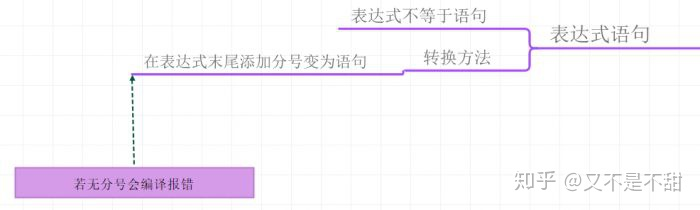图片5：表达式语句表达式和语句并不是等同的，但在编写C语言程序时，我们需要将表达式转换为语句，转换方式为在表达式后添加“；”。如果没有在其后添加分号，则会出现编译报错的现象。这些就是C语言表达式中的基础内容，下面附上完整的思维导图。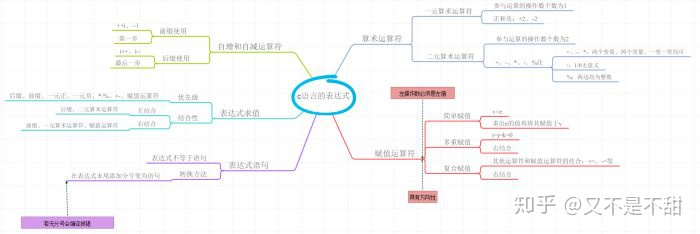图片6：C语言表达式思维导图如果您也有这样的需要，欢迎进入官网下载使用iMindMap制作属于自己的思维导图。
展开全文• 计算中缀表达式的值，用到了树，堆栈的概念，是一次上机实验作业
• c语言用栈实现计算中缀表达式 解决这个问题需要两步： 将中缀式换成后缀式（后缀式更利于写算法） 计算后缀式。 因为还要满足多位数和小数，所以用了字符串。 typedef struct { char c; }X; typedef struct ...算法
• 将由数字和四则运算符组成的后缀表达式变换为中缀表达式。 输入的后缀表达式包含的运算符不超过15个。 要求转换后的中缀表达式中不应出现不必要的括号。 例如，整个表达式两端的括号要省略，不影响原计算结果的括号...数据结构
• //存放中缀表达式原式，临时运算符 //----------- StackNum n1; StackChar c1; InitNum(&n1); InitChar(&c1); //------------ for (;;) { printf("请输入中缀表达式："); gets(str); /* 注意字符串...
• // 开始按照逆波兰计算结果 RpnNode *caclHead; caclHead = (RpnNodeList)malloc(sizeof(RpnNode)); caclHead->next = NULL; tmpStruct = (RpnNodeList)malloc(sizeof(RpnNode)); tmpStruct->next = NULL; ...
• C语言中，将中缀表达式转成后缀表达式，并计算它的结果，具体的思路如下： 1、定义两个栈，numStack用于存放运算对象，最后存放运算结果的，fuStack用于存放运算符号的 2、从左到右遍历字符串 3、如果当前的字符是...
• C语言中缀表达式 计算求值、后缀表达式 计算求值、中缀表达式 转 后缀表达式指针 c++ 数据结构
• 在开始之前，我们首先要了解以下运算符在C语言中的优先级： ...这样的一个表达式就是中缀表达式（也称中序表达式），但由于这种表达式不容易被计算机解析，所以我们需要将其转化为后缀表达式！ 后...后缀表达式
• ## C语言中缀表达式求值(综合)

千次阅读 多人点赞 2019-04-13 10:59:06
基本思路:先把输入的中缀表达式→后缀表达式→进行计算得出结果 栈：”先进先出，先进后出“！ 中缀转后缀（先把转换后的后缀表达式存入字符数组）：从左至右依次读取，遇到运算数存入字符数组，遇到运算符压入栈...C 数据结构
• #include &lt;stdio.h&gt; #include &lt;stdlib.h&gt; #include &lt;...//后缀表示法计算时候的思路！！！先把元素推到栈中，每碰到一个 char stack; char output;...c
• 中缀表达式转换为后缀表达式 关于括号，直接将括号里面的符号加入后缀表达式。 关于数字，直接推入后缀表达式 遇到±符号，如果栈为空或者栈顶为“（”，直接将符号推入栈，如果栈不为空，且栈顶不为“（”，则推出...C
• 在标准的四则混合运算表达式中(或者我们称之为中缀表达式)，遇到一个操作符是不能直接计算的，因为计算的顺序要取决于后面的运算符。多举几个例子，大家就能明白了。由于加和减是相同的运算优先级，乘和除是相同的...
• C语言一道练习如何建立栈和运用栈来进行...里面涉及加减乘除括号的优先级考虑和入栈出栈的规则来实现计算中缀表达式。作为数据结构中比较重要的一个结构——栈，我们可以通过这道题更加好的理解栈的用途并熟悉栈的运用
• 算术表达式有前缀表示法、中缀表示法和后缀表示法等形式。前缀表达式指二元运算符位于两个运算数之前，例如2+3*(7-4)+8/4的前缀表达式是：+ + 2 * 3 - 7 4 / 8 4。请设计程序计算前缀表达式的结果值。 输入格式: ...
• 利用后缀表达式计算中缀表达式的值.数据结构 花费了我2周的时间才完成的 数据结构 c语言 MFC，是用MFC做的，，该程序功能强大，健壮性很强，对于错误输入有提示，程序完全正确，解压既可以运行
• 150. 逆波兰表达式求值根据 逆波兰表示法，求表达式的值。有效的运算符包括 + , - , * , / 。每个运算对象可以是整数，也可以是另一个逆波兰表达式。说明：整数除法只保留整数部分。给定逆波...
• 从书上看到的讲起最近（半年内 ）在看《大话数据结构》，上个星期在栈这一节看到了后缀表达式用于计算的神奇力量。于是用Python试着实现了一下（功底太差，导致花了很长时间）。后缀表达式求值很容易实现，基本就是...
• 欢迎关注微信公众号：计算机二级...在逻辑表达式的求值过程中，按其操作数从左至右的计算顺序，当某个操作数的值可以确定整个逻辑表达式的值时，其余的操作数不再计算。逻辑运算符“&&”和“||”都具有短...
• 什么是表达式？计算机如何表达和计算一个算术表达式？搞懂了这个问题，我们也就弄清楚了如何去解析一...表示一个算术表达式的方法通常有三种，前缀表达式，也称为波兰表达式、中缀表达式，也就是我们人类常用的这种...lisp语言...

# c语言计算中缀表达式c语言 订阅